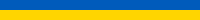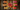# Apache Liminal: when MLOps meets GitOpsMar 31, 2021

Categories
Big Data
Containers Orchestration
Data Engineering
Data Science
Tags
Data Engineering
CI/CD
Data Science
Deep Learning
Deployment
Docker
GitOps
Kubernetes
Machine Learning
MLOps
Open source
Python
TensorFlow

Never miss our publications, subscribe to the Adaltas' newsletter about Open Source, big data and distributed systems. We maintain a low frequency of one email every two months.

Apache Liminal is an open-source software which proposes a solution to deploy end-to-end Machine Learning pipelines. Indeed it permits to centralize all the steps needed to construct Machine Learning models, from data cleaning to model deployment.

This solution proposes a declarative approach for MLOps projects. The pipeline that encapsulates the different steps for the preparation, training and deployment of your Machine Learning is written in YAML.

This file, and the Python scripts that it points to, are easily versioned using tools like Git, opening the door of a GitOps practice. GitOps describes an architecture in which the system is reproducable from the state stored in a Git repository. Data engineers and data scientists can then collaborate together to improve the model.

Apache Liminal leverages Apache Airflow, Docker and Kubernetes in oder to create and deploy our pipeline.

## Installation

To reproduce all the commands found in this article, Apache Liminal requires to install Docker and Kubernetes on your machine. Kubernetes can be installed with minikube.

If you are on MacOS with Docker already installed, the easiest approach is to activate Kubernetes by ticking the box labeled “Deploy Docker Stacks to Kubernetes by default” in Docker Desktop.

Next you can install Apache Liminal using `pip`.

``````pip install
git+https://github.com/apache/incubator-liminal.git``````

## Creation of a Liminal pipeline

### Creation of the Python scripts

Let’s start by creating a folder at the root of our project directory to gather all necessary Python scripts for our pipeline.

Inside we first create our `requirements.txt` file for dependencies management. Apache Liminal will use this file to install all the listed Python packages needed to ensure the proper functioning of our scripts on Docker images. In our example we are going to use the following packages:

``````urllib3
pandas
numpy
tensorflow
scikit-learn``````

In our use-case the data preparation step will be mainly reduced to the download of the dataset. We are going to use the wine-quality.csv file to train our model. As we will see later, these data will be directly accessible from the pods.

We are going to create a file named `download.py` that will contain all the logic to download the file and clean the data:

``````#!/usr/bin/env python

import urllib3
import pandas as pd
import numpy as np
import os

PATH = "/mnt/data/"
file_path = str(PATH) + "file.csv"

http = urllib3.PoolManager()
url = os.environ['url']

r = http.request('GET', url)

if os.path.exists(file_path):
os.remove(file_path)
else:
print("file not exist")

with open(file_path, 'xb') as f:
f.write(r.data)

for field in dataset.columns:
if type(dataset[field]) == np.int64 :
new_field = field.replace(' ', '_')
dataset = dataset.rename(columns={field : new_field})
print('i - field = ' + str(new_field))
elif type(dataset[field]) == np.float64 :
new_field = field.replace(' ', '_')
dataset = dataset.rename(columns={field : new_field})
print('f - field = ' + str(new_field))

dataset.to_csv(file_path, index=False)``````

Here we get the file using an environment variable named `url` which is defined in our YAML script as followed:

``````env_vars:
url: "https://raw.githubusercontent.com/mlflow/mlflow/master/examples/sklearn_elasticnet_wine/wine-quality.csv"``````

Next we create a python script named `wine_linear_regression.py` to train our model:

``````#!/usr/bin/env python

import os
import sys

import numpy as np
import pandas as pd
import tensorflow as tf

from six.moves import urllib
from sklearn.model_selection import train_test_split

PATH = "/mnt/data/"

path = str(PATH) + "file.csv"

labels = dataset['quality'].tolist()

dataset = dataset.drop(["quality"], axis=1)

x_train, x_test, y_train, y_test = train_test_split(dataset,
labels,
train_size=0.9)

NUMERIC_COLUMNS = ['alcohol', 'chlorides', 'citric_acid', 'density', 'fixed_acidity',
'free_sulfur_dioxide', 'pH', 'residual_sugar', 'sulphates', 'total_sulfur_dioxide',
'volatile_acidity']

CATEGORICAL_COLUMNS = ['quality']

feature_columns = []

for feature_name in NUMERIC_COLUMNS:
feature_columns.append(tf.feature_column.numeric_column(feature_name, dtype=tf.float32))

def make_input_fn(data_df, label_df, num_epochs=10, shuffle=True, batch_size=32):
def input_function():
ds = tf.data.Dataset.from_tensor_slices((dict(data_df), label_df))
if shuffle:
ds = ds.shuffle(1000)
ds = ds.batch(batch_size).repeat(num_epochs)
return ds
return input_function

train_input_fn = make_input_fn(x_train, y_train)
eval_input_fn = make_input_fn(x_test, y_test, num_epochs=1, shuffle=False)

linear_est = tf.estimator.LinearRegressor(
feature_columns=feature_columns,
model_dir=str(PATH) + "train"
)

linear_est.train(train_input_fn)

result = linear_est.evaluate(eval_input_fn)

print("--> OUTPUT = " + str(result))

inputs = {}
for feat in feature_columns:
inputs[feat.name] = tf.compat.v1.placeholder(shape=[None], dtype=feat.dtype)

print("--> INPUTS = " + str(inputs))

Finally we create a python script to compare the efficacy of the last trained model with the model running in production in order to always keep running the best model. All the code will be written in a file named `validation.py`:

``````import pandas
import random
from pathlib import Path
import tensorflow as tf
import numpy as np
import sys
import os

PATH = "/mnt/data/"

model_dir = str(PATH) + "model"
subdirs = [x for x in Path(model_dir).iterdir()
if x.is_dir() and 'temp' not in str(x)]
latest = str(sorted(subdirs)[-1])

print("--> LATEST = " + str(latest))

model_prod_dir = str(PATH) + "model_prod"
if not os.path.exists(model_prod_dir):
os.makedirs(model_prod_dir)
subdirs_prod = [x for x in Path(model_prod_dir).iterdir()
if x.is_dir() and 'temp' not in str(x)]

if not subdirs_prod:
os.rename(latest, model_prod_dir + "/" + latest.split("/")[-1])
sys.exit(0)

latest_prod = str(sorted(subdirs_prod)[-1])
print("--> PROD = " + str(latest_prod))

# Read file and select 10% of random row
randomlist = []

nb_raw = len(df)
for i in range(0, int((nb_raw/10))):
n = random.randint(0,nb_raw)
if n<nb_raw and n>=0:
randomlist.append(n)
else:

# Predict on random row

def build_predict(df, model):
res = model(chlorides=tf.constant(df['chlorides'], dtype=tf.float32, shape=1),
alcohol=tf.constant(df['alcohol'], dtype=tf.float32, shape=1),
citric_acid=tf.constant(df['citric_acid'], dtype=tf.float32, shape=1),
residual_sugar=tf.constant(df['residual_sugar'], dtype=tf.float32, shape=1),
total_sulfur_dioxide=tf.constant(df['total_sulfur_dioxide'], dtype=tf.float32, shape=1),
free_sulfur_dioxide=tf.constant(df['free_sulfur_dioxide'], dtype=tf.float32, shape=1),
pH=tf.constant(df['pH'], dtype=tf.float32, shape=1),
fixed_acidity=tf.constant(df['fixed_acidity'], dtype=tf.float32, shape=1),
sulphates=tf.constant(df['sulphates'], dtype=tf.float32, shape=1),
density=tf.constant(df['density'], dtype=tf.float32, shape=1),
# quality=tf.constant(df['quality'], dtype=tf.int64, shape=1),
volatile_acidity=tf.constant(df['volatile_acidity'], dtype=tf.float32, shape=1)
)
return res

pred = []
pred_prod = []
score_train=0
score_prod=0

## Compare prediction with the real value

for x in randomlist:
value = df.drop(["quality"], axis=1).iloc[x]
real = df['quality'].iloc[x]
pred_train = round(np.array(build_predict(value, model)['predictions']))
if real == pred_train:
score_train += 1
pred_prod = round(np.array(build_predict(value, model_prod)['predictions']))
if real == pred_prod:
score_prod += 1

print("score_train : " + str(score_train))
print("score_prod : " + str(score_prod))

## Replace new model in prod if it better than the last, Predict on random rows

if score_train > score_prod:
model_old_dir = str(PATH) + "model_old"
if not os.path.exists(model_old_dir):
os.makedirs(model_prod_dir)
os.rename(latest_prod, str(PATH) + "model_old/" + latest_prod.split("/")[-1])
os.rename(latest, model_prod_dir + "/" + latest.split("/")[-1])``````

### Creation of the pipeline

Now we are going to create a YAML file at the root of our project directory named `liminal.yml`. First let’s declare our mounting volumes. For that we create a Kubernetes volume named `data` linked to the directory where our `liminal.yml` file is located.

``````name: GettingStartedPipeline
volumes:
- volume: data
local:
path: .``````

Next we will structure and declare the ordering of our pipeline using a `tasks`. A `tasks` is composed of several `task` and is characterized by:

• `task` that is the name of the task (be careful each task has a unique name)
• `type` that specifies the type of scripts that will be run, in our case we are using Python scripts
• `description` that permits to describe the objective of the task
• `image` that specifies to which Docker images the script will be associated with
• `source` that indicates the path where the script is located
• `cmd` that allows to alias the execution command of the script
• `mounts` that allows to mount internal volume as defined above in a folder
• `env_vars` that specifies the environment variables we want to provision to our images.

Each task is run by an Airflow DAG in a distinct pod. In our case they all share the same Docker image, declared in the `image` field, and the same volume specified in the `mounts` field.

``````name: GettingStartedPipeline
volumes:
- volume: data
local:
path: .
pipelines:
- pipeline: getting_started_pipeline
owner: Aargan
start_date: 1970-01-01
timeout_minutes: 10
schedule: 0 * 1 * *
key1: val1
key2: val2
metrics:
namespace: TestNamespace
backends: [ ]
type: python
image: python_hello_world_example_image
source: pythonscript
mounts:
- mount: mymount
volume: data
path: /mnt/data
env_vars:
url: "https://raw.githubusercontent.com/mlflow/mlflow/master/examples/sklearn_elasticnet_wine/wine-quality.csv"
type: python
description: training model
image: python_hello_world_example_image
source: pythonscript
mounts:
- mount: mymount
volume: data
path: /mnt/data
cmd: python -u wine_linear_regression.py
type: python
description: validation model
image: python_hello_world_example_image
source: pythonscript
mounts:
- mount: mymount
volume: data
path: /mnt/data
cmd: python -u validation.py``````

## Run Apache Liminal

Now, let’s deploy our pipeline using the following commands:

``````liminal build
liminal deploy --clean
liminal start``````

Apache Liminal is started. The Apache Airflow UI is accessible at the following address: http://127.0.0.1:8080

Just activate the DAG and the pipeline will be triggered automatically.

We follow our DAG and access the logs through the `Tree View` (see our article Introducing Apache Airflow on AWS if you wish to better understand Apache Airflow functionalities).

Once the pipeline is fully executed and terminated we stop our Liminal server using the command:

``liminal stop``

## Conclusion

Apache Liminal proposes to simplify the creation of end-to-end Machine Learning pipelines. We think the initiative is a success. Indeed one YAML file allows you to coherently describe the execution of your different Machine Learning pipelines.

Additionally, leveraging Kubernetes let the user deploy its pipelines in remote clusters. You connect to your remote cluster using the command:

``````# Running pipeline on remote cluster has not been tested here
kubectl config set-context <your remote kubernetes cluster>``````

Finally the use of declarative YAML files presents the advantage of automating your Machine Learning pipeline in your CI/CD pipelines in order to version, publish and operate your models.

# Canada - Morocco - France

10 rue de la Kasbah
2393 Rabbat